# Arc Flash calculations according to IEEE 1584-2018 test case

Before each new release of EA-PSM Electric, validation cases are prepared, thus ensuring new features are working properly. At this validation case, arc flash scenarios for three-phase medium voltage and low voltage systems according to the IEEE 1584-2018 standard are analysed.

### System description

A simple single-line diagram to simulate an arc flash scenario in one of the busses is created. System consists of 2 busses, transmission line between them and a fuse.

#### Case 1: Vertical conductors/electrodes inside a metal box/enclosure VCBMedium-voltage system

System parameters are provided at Table 1.

Arcing currents

Intermediate average arcing currents are defined using 1.1. equation: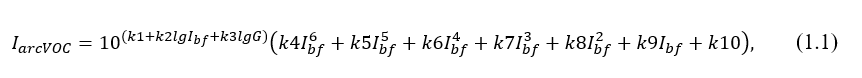where:

Ibf is the bolted fault current for three-phase faults (symmetrical rms) (kA)
Iarc_600 is the average rms arcing current at Voc = 600 V (kA);
Iarc_2700 is the average rms arcing current at Voc = 2700 V (kA);
Iarc_14300  is the average rms arcing current at Voc =14 300 V (kA);
G is the gap distance between electrodes (mm);
k1 to k10 are the coefficients provided in standard;
lg is log10.

Coefficients for the further calculations are in reference to IEEE 1584-2018. Particular values for this validation case scenarios are provided at Annex No.1 Calculation coefficients

According to IEEE 1584-2018, intermediate arcing currents for 600 V, 2700 V and 14 300 V must be calculated as follows: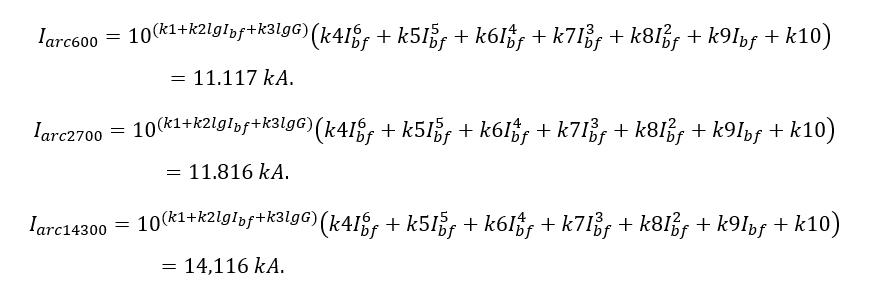Then accordingly arcing currents are calculated as follows: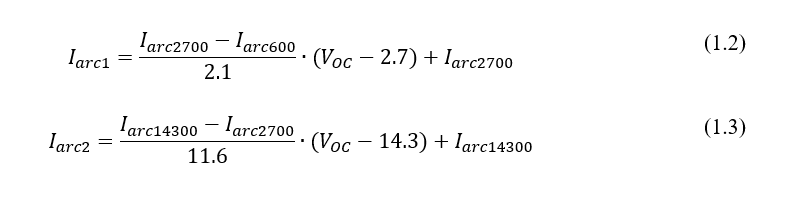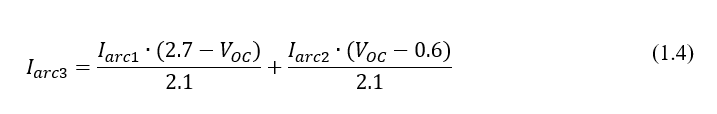Then arcing currents are: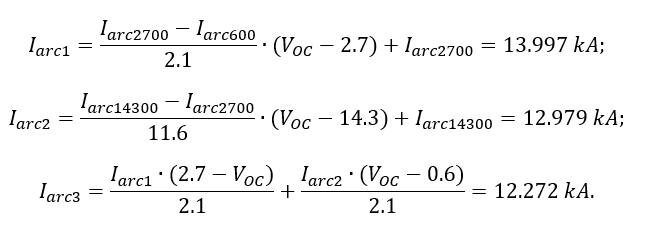The final arcing current for Voc > 2,7  is: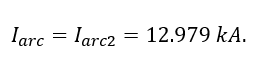Then arc duration is determined using I_arc from a power fuse time current characteristic (TCC). In this case, arc duration is: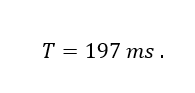Enclosure size correction factor

Based on Enclosure type (Typical) and dimensions, equivalent width is calculated: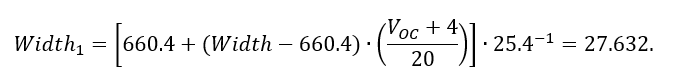Equivalent height: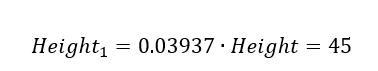Thus, equivalent enclosure size value is: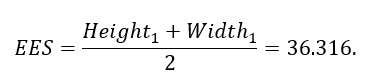The correction factor for a VCB electrode configuration is: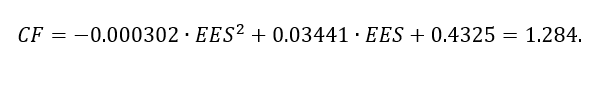Intermediate values of incident energy

Intermediate values of incident energy are calculated using coefficients that are in reference to IEEE 1584-2018.

Thus, intermediate incident energy for 600 V: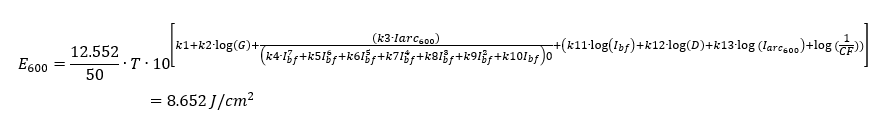Intermediate incident energy for 2700 V: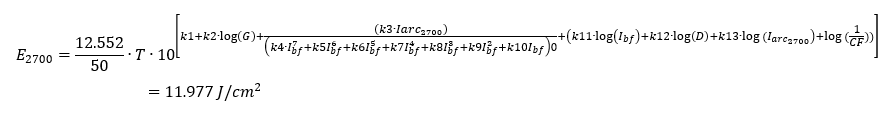Intermediate incident energy for 14300 V: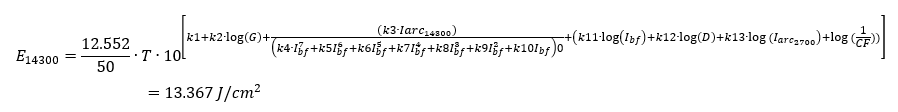The final incident energy is: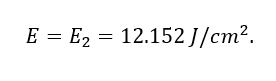Arc Flash boundary

Intermediate values of arc-flash boundary are calculated using coefficients that are in reference to IEEE 1584-2018.

Thus, intermediate arc-flash boundary for 600 V is calculated from equation: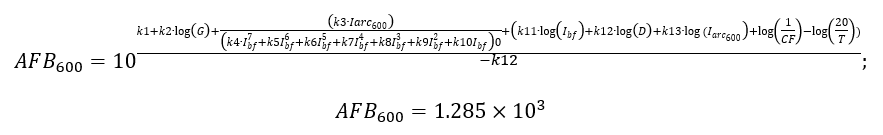Intermediate arc-flash boundary for 2700 V: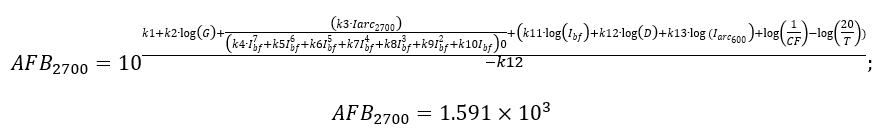Intermediate arc-flash boundary for 14300 V: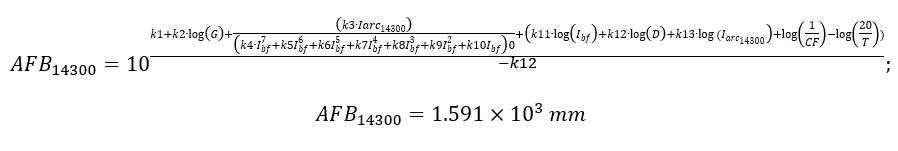The final value of arc-flash boundary is determined: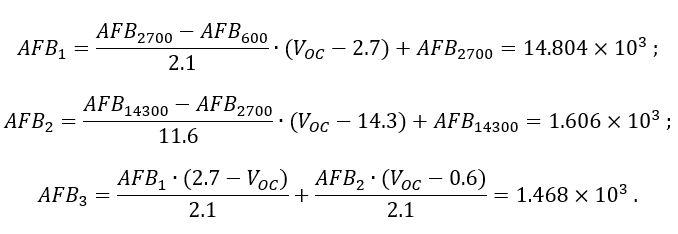The final arc-flash boundary is: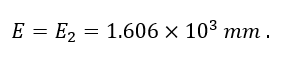## EA-PSM Calculations

Calculations using EA-PSM are performed for the test scheme that is depicted at Figure 1. Conductor configuration and enclosure parameters are defined at Bus properties (Figure 2), while protection device parameters are defined depending on device.

Calculation results are presented at the EA-PSM table and can be exported to .xlsx file format.
EA-PSM also allow to print a warning label for required safety measures such as presented at Figure 3.

## EA-PSM Calculation results: Case 1. Vertical conductors/electrodes inside a metal box/enclosure (VCB)

Results comparison between validation calculations based on IEEE 1584-2018 standard  and calculations performed using EA-PSM are depicted at Table 2.

## Conclusions

Difference between validation calculations based on IEEE Std 1584-2018 and EA-PSM calculations differ insignificantly by not more than 0.51%.

As shown by this test case, EA-PSM is a reliable tool for correctly calculating Arc flash parameters.

## References

IEEE Std 1584-2018

IEEE Guide for Performing Arc-Flash Hazard Calculations